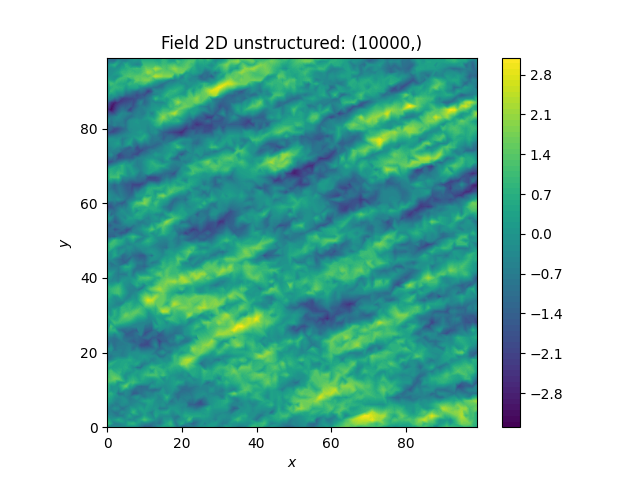# Using an Unstructured Grid¶

For many applications, the random fields are needed on an unstructured grid. Normally, such a grid would be read in, but we can simply generate one and then create a random field at those coordinates.

```import numpy as np
import gstools as gs
```

Creating our own unstructured grid

```seed = gs.random.MasterRNG(19970221)
rng = np.random.RandomState(seed())
x = rng.randint(0, 100, size=10000)
y = rng.randint(0, 100, size=10000)

model = gs.Exponential(dim=2, var=1, len_scale=[12, 3], angles=np.pi / 8)
srf = gs.SRF(model, seed=20170519)
field = srf((x, y))
srf.vtk_export("field")
# Or create a PyVista dataset
# mesh = srf.to_pyvista()
```
```ax = srf.plot()
ax.set_aspect("equal")
```Comparing this image to the previous one, you can see that be using the same seed, the same field can be computed on different grids.

Total running time of the script: ( 0 minutes 1.615 seconds)

Gallery generated by Sphinx-Gallery